# 用于 MQL5 向导的 NRTR 指标和交易模块

8 一月 2018, 09:01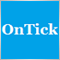0
6 270

### NRTR 指标

NRTR (Nick Rypock Trailing Reverse) 指标是由 Konstantin Kopyrkin 提出的。有趣的信息: 尼克·雷普克 (Nick Rypock) 这个名字源自姓氏 Kopyrkin。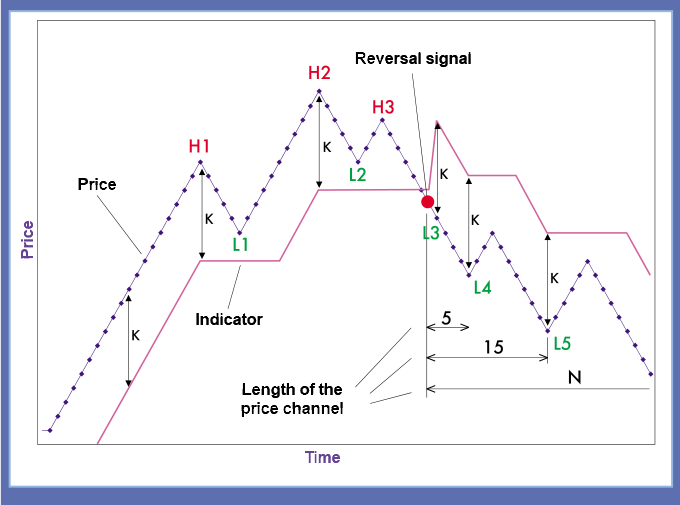### 编写指标: 从简单到复杂

```#property indicator_chart_window
#property indicator_buffers 4
#property indicator_plots   4

//指标线条样式
#property indicator_type1  DRAW_LINE
#property indicator_color1 Green
#property indicator_style1 STYLE_DASH

#property indicator_type2  DRAW_LINE
#property indicator_color2 Red
#property indicator_style2 STYLE_DASH

#property indicator_type3  DRAW_ARROW
#property indicator_color3 Green

#property indicator_type4  DRAW_ARROW
#property indicator_color4 Red

```

```input int    period =12;      //动态周期
input double percent =0.2;    //缩进百分比

double Buff_Up[],Buff_Dn[];
double Sign_Up[],Sign_Dn[];

```

```   SetIndexBuffer(2,Sign_Up,INDICATOR_DATA);
PlotIndexSetDouble(2,PLOT_EMPTY_VALUE,0.0);
PlotIndexSetInteger(2,PLOT_ARROW,236);
PlotIndexSetInteger(2,PLOT_LINE_WIDTH,1);
ArraySetAsSeries(Sign_Up,true);

```

```//+------------------------------------------------------------------+
//| 自定义指标迭代函数                                                  |
//+------------------------------------------------------------------+
int OnCalculate(const int rates_total,
const int prev_calculated,
const datetime &time[],
const double &open[],
const double &high[],
const double &low[],
const double &close[],
const long &tick_volume[],
const long &volume[],
{

int start =0;                                           //计算的起始点

int trend =0;                                           //趋势值, 0 向上 -1 向下
static int trend_prev =0;

double value =0;                                        //指标值
static double value_prev =0;

int dyn_period =1;                                     //动态周期值
static int curr_period =1;

double maxmin =0;                                       //用于计算的技术变量

ArraySetAsSeries(close,true);

if(rates_total<period) return(0);

if(prev_calculated==0)                              // 检查指标计算的首次开始
{
start=rates_total-1;                               // 所有柱线的起始计算索引
trend_prev =1;
value=close[start]*(1-0.01*percent);
}

else
{
start=rates_total-prev_calculated;                  // 新柱线的起始计算索引
}

trend =trend_prev;
value =value_prev;
dyn_period =curr_period;

```

```trend =trend_prev;
value=value_prev;
dyn_period =curr_period;
//-------------------------------------------------------------------+
//                        主要的计算循环
//-------------------------------------------------------------------+
for(int i=start;i>=0;i--)
{
Buff_Up[i] =0.0;
Buff_Dn[i] =0.0;
Sign_Up[i] =0.0;
Sign_Dn[i] =0.0;

if(curr_period>period) curr_period=period;
if(dyn_period>period) dyn_period=period;

// 如果趋势为升势
if(trend>0)
{
maxmin =close[ArrayMaximum(close,i,dyn_period)];
value =maxmin*(1-percent*0.01);

if(close[i]<value)
{
maxmin =close[i];
value =maxmin*(1+percent*0.01);
trend =-1;
dyn_period =1;
}
}

// 如果趋势为降势
else
{
maxmin =close[ArrayMinimum(close,i,dyn_period)];
value =maxmin*(1+percent*0.01);
if(close[i]>value)
{
maxmin =close[i];
value =maxmin*(1-percent*0.01);
trend =1;
dyn_period =1;
}
}
// 趋势变化

if(trend>0) Buff_Up[i] =value;
if(trend<0) Buff_Dn[i] =value;

if(trend_prev<0  &&  trend>0)
{
Sign_Up[i] =value;
Buff_Up[i] =0.0;
}
if(trend_prev>0 && trend<0)
{
Sign_Dn[i] =value;
Buff_Dn[i] =0.0;
}

dyn_period++;

if(i)
{
trend_prev =trend;
value_prev =value;
if(dyn_period==2)curr_period =2;
else curr_period++;
}

}

```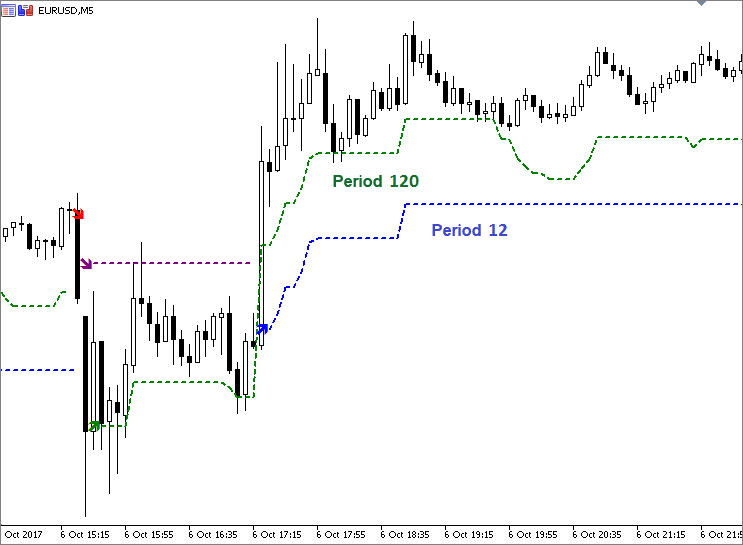### 波动性和 NRTR

`handle_atr =iATR(_Symbol,PERIOD_CURRENT,period);`

ATR 周期可以不同于有效的动态周期。一个合乎逻辑的解决方案是令它们相等, 这样的话参数数目不会改变。

```#property indicator_buffers 5
#property indicator_plots   4
.............................
input double K =1;            //缩放系数
double Buff_ATR[];
int handle_atr;
.............................
SetIndexBuffer(4,Buff_ATR,INDICATOR_CALCULATIONS);
ArraySetAsSeries(Buff_ATR,true);

handle_atr =iATR(_Symbol,PERIOD_CURRENT,period);
.....................................................
int OnCalculate(){
.....................................................
if(CopyBuffer(handle_atr,0,0,start+1,Buff_ATR)==-1)
{
return(0);
Print("复制数据到 ATR 缓冲区失败");
}
.....................................................

// 如果趋势为升势
if(trend>=0)
{
maxmin =close[ArrayMaximum(close,i,dyn_period)];
value =maxmin-K*Buff_ATR[i];

if(close[i]<value)
{
maxmin =close[i];
value =maxmin+K*Buff_ATR[i];
trend =-1;
dyn_period =1;
}
}

}

```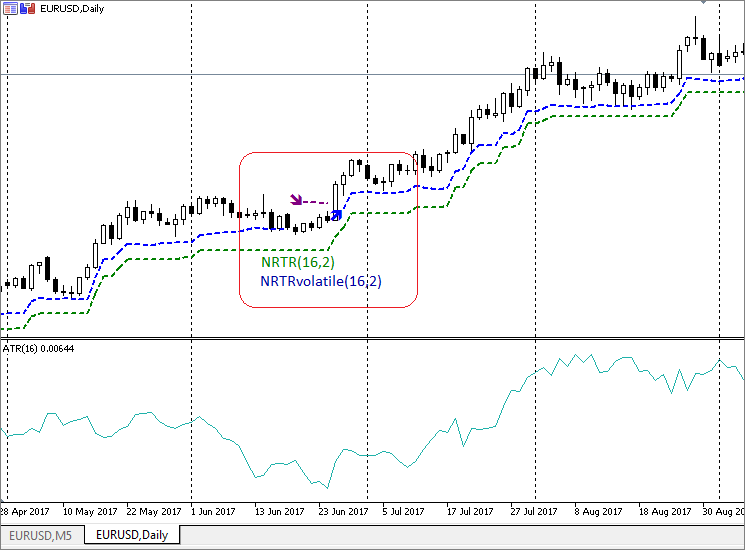### 用于 MQL5 向导的交易模块

• 模块描述符
• 交易参数和参数初始化函数
• 检查输入参数
• 将选定的指标与模块连接
• 交易策略的描述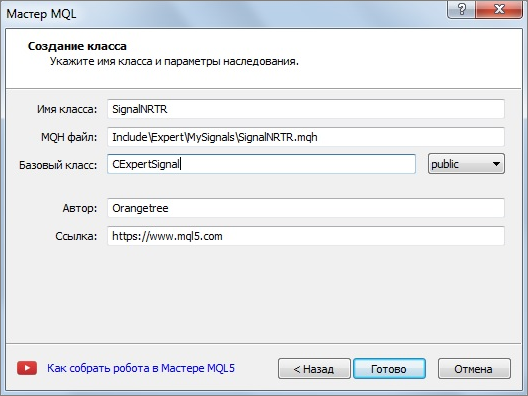CExpertSignal 基类的路径添加到向导生成的代码里: #include "..\ExpertSignal.mqh"

```//+------------------------------------------------------------------+
//|                                                   SignalNRTR.mqh |
//|                                                       Orangetree |
//|                                             https://www.mql5.com |
//+------------------------------------------------------------------+
#property version   "1.00"

#include "..\ExpertSignal.mqh"              // CExpertSignal 类
//+------------------------------------------------------------------+
//|                                                                  |
//+------------------------------------------------------------------+
class SignalNRTR : public CExpertSignal
{
private:

public:
SignalNRTR();
~SignalNRTR();
};
//+------------------------------------------------------------------+
//|                                                                  |
//+------------------------------------------------------------------+
SignalNRTR::SignalNRTR()
{
}
//+------------------------------------------------------------------+
//|                                                                  |
//+------------------------------------------------------------------+
SignalNRTR::~SignalNRTR()
{
}
//+------------------------------------------------------------------+

```

```// wizard description start
//+------------------------------------------------------------------+
//| Description of the class                                         |
//| Title=Signals of indicator 'NRTR'                                |
//| Name=NRTR                                                        |
//| ShortName=NRTR                                                   |
//| Class=SignalNRTR                                                 |
//| Page=????                                                        |
//| Parameter=PeriodDyn,int,12,Dynamic channel period                |
//| Parameter=PercentDev,double,0.1,Channel width in percent         |
//+------------------------------------------------------------------+
// wizard description end
//+------------------------------------------------------------------+
//| Class SignalNRTR.                                                |
//| Purpose: Class of generator of trade signals based on            |
//|          the 'NRTR' indicator.                                   |
//| Is derived from the CExpertSignal class.                         |
//+------------------------------------------------------------------+

```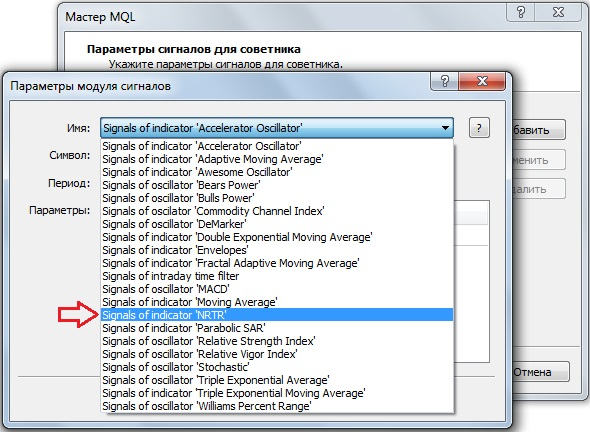```class SignalNRTR : public CExpertSignal
{
protected:
int m_period_dyn;                                   // 通道周期
double m_percent_dev;           // 通道宽度为价格的百分比

public:
SignalNRTR();
~SignalNRTR();
//--- 设置可调参数的方法
void              PeriodDyn(int value)                 { m_period_dyn=value;}
void              PercentDev(double value)             { m_percent_dev=value;}

};
//+------------------------------------------------------------------+
//|                                                                  |
//+------------------------------------------------------------------+
SignalNRTR::SignalNRTR() : m_period_dyn(12),
m_percent_dev(0.1)
{
//--- 受保护数据的初始化
m_used_series=USE_SERIES_OPEN+USE_SERIES_HIGH+USE_SERIES_LOW+USE_SERIES_CLOSE;
}

```

CExpertBase 类中的虚方法 bool ValidationSettings() 能够检查输入的正确性。

```//+------------------------------------------------------------------+
//| 检查输入参数的方法                                                  |
//+------------------------------------------------------------------+
bool SignalNRTR:: ValidationSettings()
{
// 调用基类方法
if(!CExpertSignal::ValidationSettings())  return(false);

// 周期必须大于 1
if(m_period_dyn<2)
{
Print(周期必须大于 1);
return false;
}
// 通道宽度值必须是正数值
if(m_percent_dev<=0)
{
Print("通道宽度值必须是正数值");
return false;
}

return true;
}

```

```//+------------------------------------------------------------------+
//| 创建指标。                                                         |
//+------------------------------------------------------------------+
bool SignalNRTR::InitIndicators(CIndicators *indicators)
{
//--- 检查指针
if(indicators==NULL)
return(false);
//--- 初始化指标和时间序列的额外过滤器
if(!CExpertSignal::InitIndicators(indicators))
return(false);
//--- 创建并初始化 NRTR 指标
if(!InitNRTR(indicators))
return(false);
//--- ok
return(true);
}

```

```//+------------------------------------------------------------------+
//| 创建 NRTR 指标。                                                   |
//+------------------------------------------------------------------+
bool SignalNRTR::InitNRTR(CIndicators *indicators)
{
//--- 检查指针
if(indicators==NULL)
return(false);
//--- 将对象加入集合
{
printf(__FUNCTION__+": 添加对象出错");
return(false);
}
//--- 设置 NRTR 参数
MqlParam parameters;
//---
parameters.type=TYPE_STRING;
parameters.string_value="Orangetree\\NRTR.ex5";
parameters.type=TYPE_INT;
parameters.integer_value=m_period_dyn;      // 周期
parameters.type=TYPE_DOUBLE;
parameters.double_value=m_percent_dev;      // 通道宽度
//--- 初始化对象
if(!m_nrtr.Create(m_symbol.Name(),m_period,IND_CUSTOM,3,parameters))
{
printf(__FUNCTION__+": 初始化对象出错");
return(false);
}
//--- ok
return(true);
}

```

```   //--- 获取数据的方法
double            UpSignal(int index)                   { return(m_nrtr.GetData(2,index));}
double            DnSignal(int index)                   { return(m_nrtr.GetData(3,index));}

```

GetData() 函数根据其索引和柱线索引接收指标缓冲区的数值。指标已包含了趋势反转信号。这就是为什么开仓的条件很简单。如果指标产生 "上升" 信号, 我们买入, 如果产生 "下行" 信号, 则卖出。

```//+------------------------------------------------------------------+
//| "投票", 价格会增长。                                                |
//+------------------------------------------------------------------+
int SignalNRTR::LongCondition(void)
{
int idx   =StartIndex();
if(UpSignal(idx))
return 100;
else return 0;
}
//+------------------------------------------------------------------+
//| "投票", 价格会下跌。                                                |
//+------------------------------------------------------------------+
int SignalNRTR::ShortCondition(void)
{
int idx   =StartIndex();
if(DnSignal(idx))
return 100;
else return 0;
}

```

virtual int StartIndex() 函数的描述包含以下语句: "如果分析当前柱线的标志设置为 true (从当前柱线分析), 则方法返回 0。如果没有设置标志, 它将返回 1 (从最后一根完成的柱线分析)。"我们的系统只分析完整柱线上的信号。按照我们的策略 StartIndex() 函数默认返回 1。此功能在智能交易系统中通过 Expert_EveryTick 参数设为 false 来反映。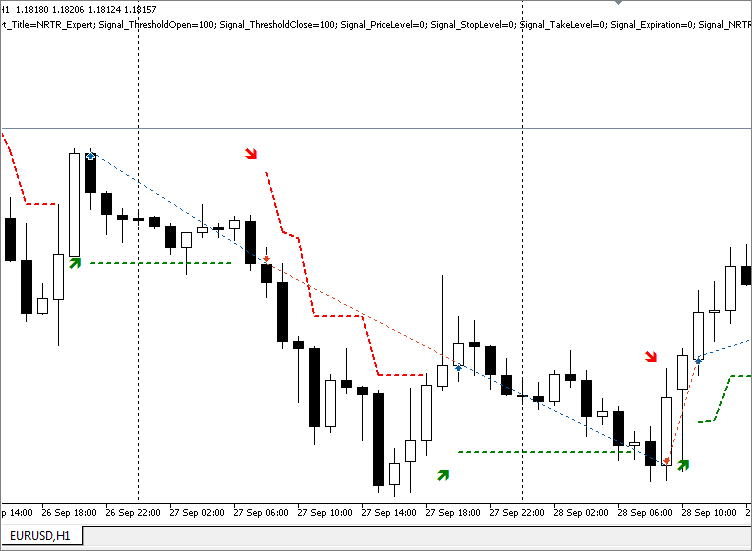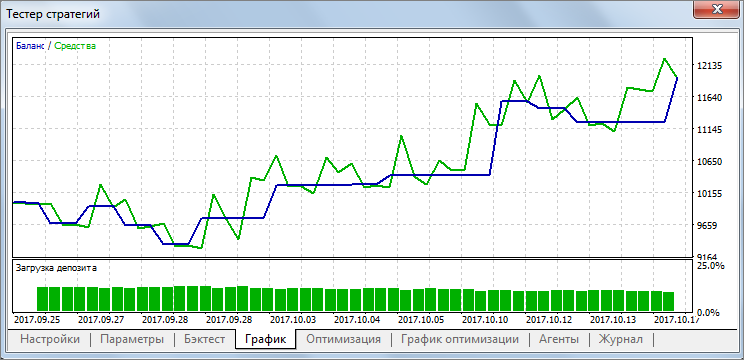### NRTR 与不同趋势指标的结合

```protected:
....................... 其它代码.....................................

//--- 初始化对象
{
printf(__FUNCTION__+": 初始化对象出错");
return(false);
}

```

```//| Parameter=PeriodADX,int,14,ADX indicator period
.....................................................

//--- 设置可调参数的方法
.....................................................

//--- 获取数据的方法

```

• 买入, 如果 +DI >-DI 且 ADX 增长。
• 卖出, 如果 +DI <-DI 且 ADX 增长。
```//+------------------------------------------------------------------+
//| "投票" 这种趋势是 "向下"。                                          |
//+------------------------------------------------------------------+
{
int idx   =StartIndex();
return (100);
else
return (0);
}
//+------------------------------------------------------------------+
//| "投票" 这种趋势是 "向上"。                                          |
//+------------------------------------------------------------------+
{
int idx   =StartIndex();
return (100);
else
return (0);
}

```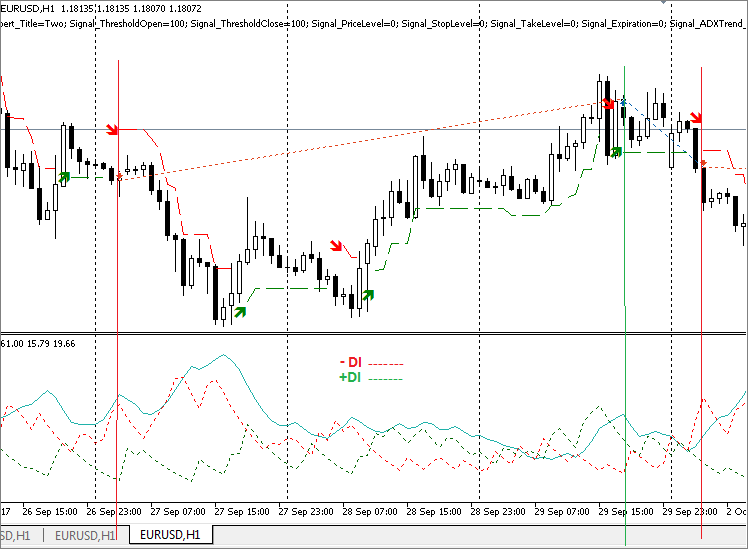### 结束语

`   parameters.string_value="NRTR.ex5";`

#名称类型描述
1NRTR.mq5指标所分析的指标源代码
2NRTRvolatile.mq5指标价格波动性指标的源代码
3SignalNRTR.mqh交易模块交易信号模块。它用于在 MQL5 向导中生成智能交易系统

MQL5.zip 文件夹中的文件根据 MetaEditor 目录进行定位。

NRTR.mq5 (11.09 KB)
NRTRvolatile.mq5 (11.93 KB)
SignalNRTR.mqh (14.21 KB)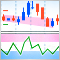动量弹球交易策略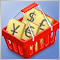测试当交易货币对篮子时出现的形态第二部分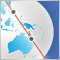在亚洲市场进行夜间交易: 如何保持盈利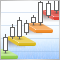利用迪纳波利 (DiNapoli) 等级进行交易